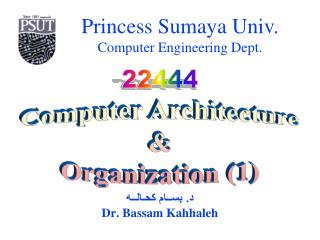DownloadDownload Presentationد. بســام كحـالــه Dr. Bassam Kahhaleh

# د. بســام كحـالــه Dr. Bassam Kahhaleh

Download Presentation## د. بســام كحـالــه Dr. Bassam Kahhaleh

- - - - - - - - - - - - - - - - - - - - - - - - - - - E N D - - - - - - - - - - - - - - - - - - - - - - - - - - -
##### Presentation Transcript

1. د. بســام كحـالــه Dr. Bassam Kahhaleh 22444 Computer Architecture&Organization (1)

2. “Mano” Chapter 4: 22444 - Computer Architecture & Organization (1) Register Transfer & Microoperations

3. Micro-Operations A micro-operation is an elementary operation, performed during one clock pulse, on the information stored in one or more registers. R1 ← R1 + R2

4. Register Designation • Whole register • Portion of a register • One bit in a register

5. Parallel Register Transfer • Unconditional R1 ← R2 • Conditional P: R1 ← R2 • Simultaneous R1 ← R2 , R3 ← R2

6. Bus Transfer R2 ← RA R1 ← RD Consider: Ri ← Rx where i = 0, 1, 2 or 3 x = A, B, C or D

7. Bus Transfer

8. Bus Transfer

9. Bus Transfer

10. Bus Transfer

11. Bus Transfer

12. Bus Transfer

13. Micro-Operation Types • Data Transfer • Arithmetic Operations • Logic Operations • Shift Operations

14. Micro-Operation Types • Data Transfer • Arithmetic Operations S = A + B • Logic Operations • Shift Operations

15. Addition S = A + B Time (Propagation) delay = ?

16. Addition S = A + B t = 0 Time (Propagation) delay = ?

17. Addition S = A + B t = t Time (Propagation) delay = ?

18. Addition S = A + B t = 2t Time (Propagation) delay = ?

19. Addition S = A + B t = 3t Time (Propagation) delay = ?

20. Addition S = A + B t = 4t Time (Propagation) delay = 4 t

21. Addition A ← A + B Flag

22. Subtraction A ← A⎯B _ A ← A + ( B + 1 )

23. Increment A ← A + 1

24. Decrement A ← A⎯ 1

25. Arithmetic

26. Arithmetic

27. Arithmetic

28. Arithmetic Data Control

29. Micro-Operation Types • Data Transfer • Arithmetic Operations • Logic Operations • Shift Operations AND: S = AΛB OR: S = A V B XOR: S = AB AND: S = A • B OR: S = A + B XOR: S = AB

30. Logic

31. Logic

32. Logic

33. Logic

34. Logic Data Control

35. Micro-Operation Types • Data Transfer • Arithmetic Operations • Logic Operations • Shift Operations • Logical Shift shl A shr A • Arithmetic Shift ashl A ashr A • Circular Shift cil A cir A

36. Logical & Arithmetic Shift

37. Logical & Arithmetic Shift

38. Logical & Arithmetic Shift

39. Logical & Arithmetic Shift

40. Logical & Arithmetic Shift

41. Logical, Arithmetic, & Circular Shift

42. Logical, Arithmetic, & Circular Shift

43. Arithmetic and Logic Unit (ALU)

44. Chapter 4 4-1 4-2 4-3 4-4 4-5 4-6 4-8 4-9 4-10 4-11 4-13 4-15 4-16 4-17 Homework

45. Homework • Mano

46. Homework

47. Homework

48. Homework

49. Homework

50. Homework